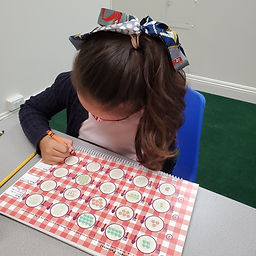## Ms. Raejean

### Target 1​

###### Lesson Type:

New

Algebra

:

Sequence

Use arithmetic to define a sequence and calculate the next unit in a series.

###### 1:

Study a given arithmetic sequence to determine what rule explains the sequence (i.e., 2-4-6-8-10…each number is the sequence gets bigger by 2).

###### 2:

Write an arithmetic rule to explain a given sequence.

###### 3:

Understand that a pattern can be described by stating the rule that it is following.

2nd

###### Vocabulary:

Sequence, Pattern, Rule

Activities:

Students worked on identifying paterns in a sequence.

Example - The plate of cookies have 3, 5, 7, __, __. Students needed to identify the pattern - The number of cookies changed by adding 2 more cookies. Then students continued the pattern. The last 2 plates have 9 and 11 cookies. Next students wrote the rule - Add 2. (or start with 3, add 2)### Home Exploration

###### Guiding Questions:## Absent Students:

### Target 2

:

###### 1:

Solve problems that have more than one operation, addition and subtraction.

###### 2:

Understand when solving a problem that has both addition and subtraction operations that solving starts on the left and continues to the right.

###### Vocabulary:

Multi-Step, Addition, Subtraction, Equation, Equal, Unknown Numbers

Activities:

• Students solved multi-step addition and subtraction equations. Example: 11 + 15 + 13 - ? = 27
• We discussed how problems need to be solved from left to right. Example: First solve 11 + 15 + 13 = 39
• We talked about the (?) or unknown number. Example: 39 - ? = 27
• We discussed how the equal sign in an equation means that the left and right side need to be equal. Example: Solve the equation with 39 - 27 = ?

*Students used base ten blocks and hundreds charts to help with adding and subtracting. We will continue to practice solving multi-step equations and solving for the unknown number. We will also work on subtraction with regrouping.### Home Exploration

###### Guiding Questions:### Target 3

:

###### Vocabulary:

Activities:### Home Exploration